RS Aggarwal Class 6 Solutions Chapter 14 - Constructions

RS Aggarwal Class 6 Chapter 14 - Constructions Solutions Free PDF

Constructions is one of the major concepts of mathematics. This chapter deals with teaching students about the basic of constructions like, how to construct a circle using a compass, how to bisect lines using compass and lot more. Solve questions on constructions using RS Aggarwal for class 6. To help students further we have also provided the RS Aggarwal class 6 solutions chapter 14 constructions.

Constructions Exercise 14.1

Question 1:

Draw a line segment PQ = 6.2 cm. Draw the perpendicular bisector of PQ.

Solution:

Steps for construction:

1. Draw a line segment PQ, which is equal to 6.2 cm.

2. With P as the centre and radius more than half of PQ, draw arcs, one on each side of PQ.

3. With Q as the centre and the same radius as before, draw arcs cutting the previously drawn arcs at A and B, respectively.

4. Draw AB, meeting PQ at R.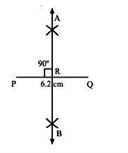Question 2:

Draw a line segment AB = 5.6 cm. Draw the perpendicular bisector of AB.

Solution:

Steps for construction:

1. Draw a line segment AB = 5.6 cm.

2. With A as the centre and radius more than half of AB, draw arcs, one on each side of AB.

3. With B as the centre and the same radius as before, draw arcs cutting the previously drawn arcs at P and Q, respectively.

4. Draw PQ, meeting AB at R.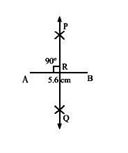Question 3:

Draw an angle equal to$\angle$AOB, given in the adjoining figure.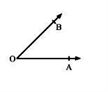Solution:

Here $\angle$AOB is given.

Steps for construction:

1. Draw a ray QP.

2. With O as the centre and any suitable radius, draw an arc cutting OA and OB at C and E, respectively.

3. With Q as the centre and the same radius as in step (2), draw an arc cutting QP at D.

4. With D as the centre and radius equal to CE, cut the arc through D at F.

5. Draw QF and produce it to point R.

Therefore, $\angle$PQR = $\angle$AOB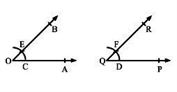Question 4:

Draw an angle of 50o with the help of a protractor. Draw a ray bisecting this angle.

Solution:

Steps for construction: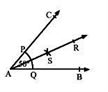1. Draw $\angle$BAC = 50o with the help of protractor.

2. With A as the centre and any convenient radius, draw an arc cutting AB and AC at Q and P, respectively.

3. With P as the centre and radius more than half of PQ, draw an arc.

4. With Q as the centre and the same radius as before, draw another arc cutting the previously drawn arc at a point S.

5. Draw SA and produce it to point R.

Then, ray AR bisects $\angle$BAC.

Question 5:

Construct $\angle$AOB = 85o with the help of a protractor. Draw a ray OX bisecting $\angle$AOB.

Solution:

Steps for construction: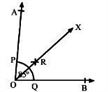1. Draw $\angle$AOB = 85o with the help of a protractor.

2. With O as the centre and any convenient radius, draw an arc cutting OA and OB at P and Q, respectively.

3. With P as the centre and radius more than half of PQ, draw an arc.

4. With Q as the centre and the same radius as before, draw another arc cutting the previously drawn arc at a point R.

5. Draw RO and produce it to point X.

Then, ray OX bisects $\angle$AOB.

Question 6:

Draw a line AB. Take a point P on it. Draw a line passing through P and perpendicular to AB.

Solution:

Steps for construction: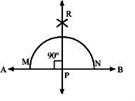1. Draw a line AB.

2. Take a point P on the line AB.

3. With P as the centre, draw an arc of any radius, which intersects line AB at M and N, respectively.

4. With M as the centre and radius more than half of MN, draw an arc.

5. With N as the centre and the same radius as in step (4), draw an arc that cuts the previously drawn arc at R.

6. Draw PR.

PR is the required line, which is perpendicular to AB.

Question 7:

Draw a line AB. Take a point P outside it. Draw a line passing through P and perpendicular to AB.

Solution:

Steps for construction: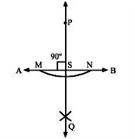1. Draw a line AB.

2. Take a point P outside AB.

3. With P as the centre and a convenient radius, draw an arc intersecting AB at M and N, respectively.

4. With M as the centre and radius more than half of MN, draw an arc.

5. With N as the centre and the same radius, draw an arc cutting the previously drawn arc at Q.

6. Draw PQ meeting AB at S.

PQ is the required line that passes through P and is perpendicular to AB.

Question 8:

Draw a line AB. Take a point P outside it. Draw a line passing through P and parallel to AB.

Solution:

Steps for construction: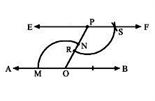1. Draw a line AB.

2. Take a point P outside AB and another point O on AB.

3. Draw PO.

4. Draw $\angle$ FPO such that $\angle$FPO is equal to AOP.

5. Extend FP to E.

Then, the line EF passes through the point P and EF || AB.

Question 9:

Draw $\angle$ABC of measure 60o such that AB = 4.5 cm and BC = 5 cm. Through C draw a line parallel to AB and through B draw a line parallel to AC, intersecting each other at D. Measure BD and CD.

Solution:

Steps for construction: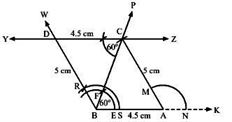1. Draw a line BX and take a point A, such that AB is equal to 4.5 cm.

2. Draw $\angle$ABP = 60o with the help of protractor.

3. With A as the centre and a radius of 5 cm, draw an arc cutting PB at C.

4. Draw AC.

5. Now, draw $\angle$BCY = 60o.

6. Then, draw $\angle$ABW, such that $\angle$ABW is equal to $\angle$CCAX, which cut the ray CY at D.

7. Draw BD.

When we measure BD and CD, we have:

BD = 5 cm and CD = 4.5 cm

Question 10:

Draw a line segment AB = 6 cm. Take a point C on AB such that AC = 2.5 cm. Draw CD perpendicular to AB.

Solution:

Steps of construction: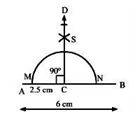1. Draw a line segment AB, which is equal to 6 cm.

2. Take a point C on AB such that AC is equal to 2.5 cm.

3. With C as the centre, draw an arc cutting AB at M and N.

4. With M as the centre and radius more than half of MN, draw an arc.

5. With N as the centre and the same radius as before, draw another arc cutting the previously drawn arc at S.

6. Draw SC and produce it to D.

Question 11:

Draw a line segment AB = 5.6 cm. Draw the right bisector of AB.

Solution:

Steps for construction: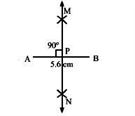1. Draw a line segment AB, which is equal to 5.6 cm.

2. With A as the centre and radius more than half of AB, draw arcs, one on each side of AB.

3. With B as the centre and the same radius as before, draw arcs cutting the previously drawn arcs at M and N, respectively.

4. Draw MN, meeting AB at R.

Practise This Question

Which change occurs during evaporation?